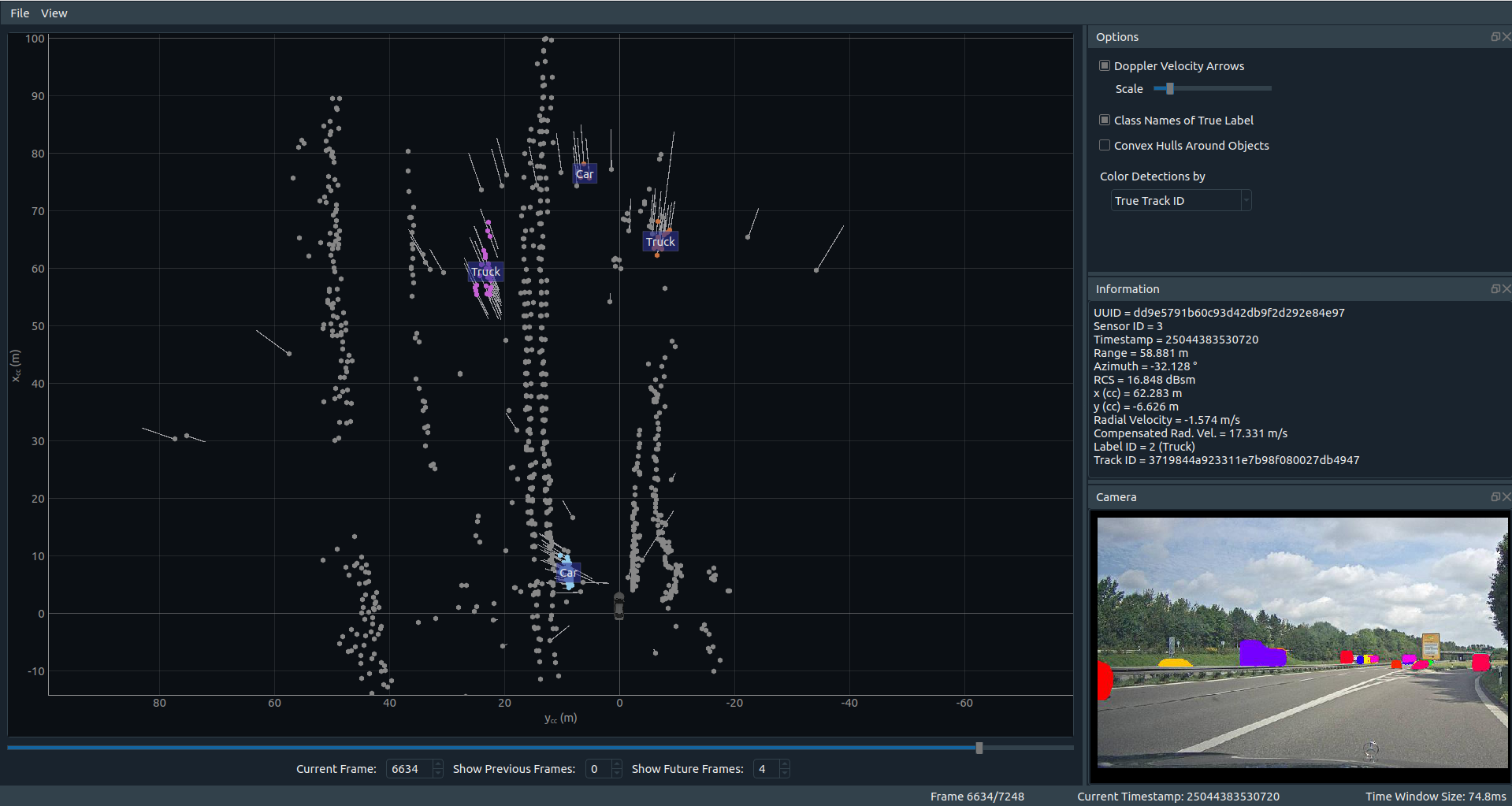Working with radar data is quite different from working with camera or lidar data. To make the start easier, we created some tools to help you.

# Viewer

You can find a viewer for the RadarScenes data set on pypi and on github.To install the viewer, simply follow the instructions on the linked pages. You will end up with a python package called `radar_scenes` which contains the viewer.

# API

Additionally, the linked `radar_scenes` python packge contains also a small API for the data set. It allows to iterate over the data and contains helper functions for example for coordinate transformations.

Example code can be found in the examples folder in the `radar_scenes` package: Github.

In future, helper functions for score calculation of different machine learning approaches will be integrated.

Feel free to contribute via pull requests!

## Label Statistics

Here is one example on how the data can be accessed: Let’s find out how many different labeled detections are contained within one sequence.

First, let’s import the necessary packages, define the path to the data set and select one sequence:

``````import os
import numpy as np
from collections import Counter

filename = os.path.join(path_to_dataset, "data", "sequence_137", "scenes.json")
``````

Then, we create a `Sequence` object for the selected sequence:

``````sequence = Sequence.from_json(filename)
``````

With this sequence object, we have easy access to the radar data. We can for example select the column containing the label ids of all detections:

``````all_labels = sequence.radar_data["label_id"]
``````

You can find the column names by either looking directly into the hdf5 files with a proper viewer or by printing out the `dtype` of the numpy array `sequence.radar_data`.

Using the `Counter` class from python’s `collections` module, we can quickly count the occurances of all labels:

``````c = Counter(all_labels)
for label_id, n in c.items():
print("In the whole sequence, class {} occurred {} times".format(Label.label_id_to_name(label_id), n))
``````

If we were interested in the number of unique objects, in each scene, we could get them as follows. First, we define a small helper function

``````def count_unique_objects(sequence: Sequence):
"""
For each scene in the sequence, count how many different objects exist.
Objects labeled as "clutter" are excluded from the counting, as well as the static detections.
:param sequence: A measurement sequence
:return: a list holding the number of unique objects for each scene
"""
objects_per_scene = []
for scene in sequence.scenes():

valid_idx = np.where(label_ids != Label.STATIC.value)
unique_tracks = set(track_ids[valid_idx])
objects_per_scene.append(len(unique_tracks))
return objects_per_scene
``````

and then call it for our sequence:

``````print("\nCounting the number of unique dynamic objects in each scene:")
object_counts = count_unique_objects(sequence)
print("The most unique objects appear in scene {} in which {} different objects were labeled.".format(np.argmax(object_counts), object_counts[np.argmax(object_counts)]))
``````

The full example contains even more hints on how to use the data set.

Make sure to check out the other examples!

Updated: